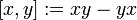# Lazard Lie ring of adjoint group of a radical ring equals associated Lie ring of the radical ring under suitable nilpotency assumptions

Suppose$N$ is a radical ring such that there exists a positive integer$n$ so that the additive group of$N$ has powering threshold at least$n - 1$ and$x^n = 0$ for all$x \in N$. Suppose$N$ has adjoint group$G$. Suppose that$G$ is a Lazard Lie group. Then, the Lazard Lie ring of$G$ is isomorphic to the Lie ring associated to$N$, i.e., the Lie ring whose additive group is the same as that of$N$ and whose Lie bracket is the additive commutator in$N$, i.e.,$[x,y] := xy - yx$.
Suppose$q$ is a power of a prime$p$ and$N$ is a nilpotent associative algebra over the finite field$\mathbb{F}_q$. Suppose, further, that$x^p = 0$ for all$x \in N$.
Let$G$ be the algebra group corresponding to$N$. Then,$G$ is a Lazard Lie group. Then, the Lazard Lie ring of$G$ is isomorphic to the Lie ring associated to$N$, i.e., the Lie ring whose additive group is the same as that of$N$ and whose Lie bracket is the additive commutator in$N$, i.e.,$[x,y] := xy - yx$.